﻿ 2019年中级财务管理经典题解（十二）_中级会计师考试 _ 233网校

233网校 中级会计师考试

2019年中级会计《财务管理》经典题解（十二）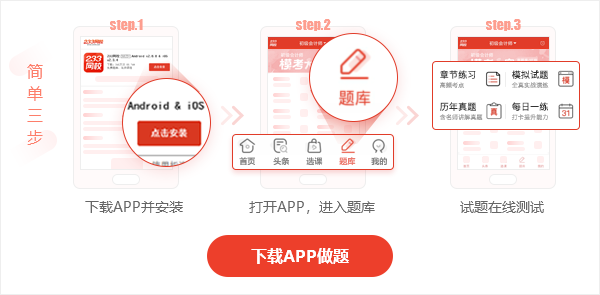2019年中级会计财务管理经典题解十二

【解析一】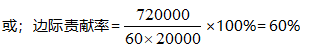【解析二】

1. 加权平均法。

2. 从表-2中第⑦栏可知，A、B、C三种产品边际贡献率分别为40%、20%和30%。

1.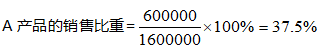2.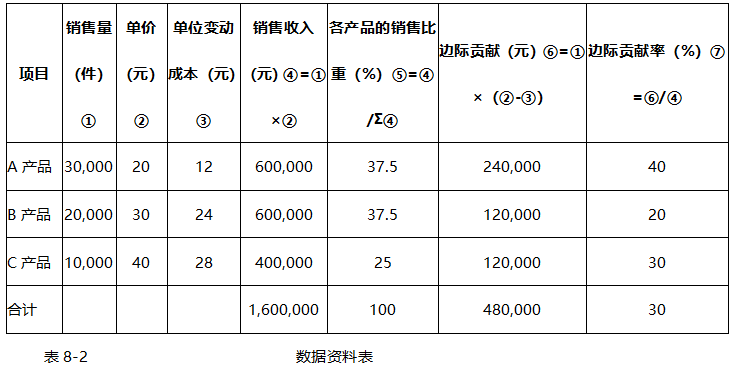3.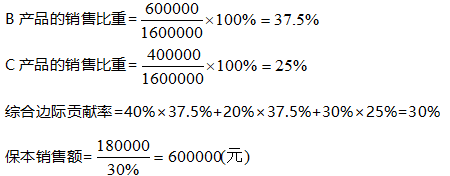4. A产品保本销售额=600 000×37.5%=225 000（元）

B产品保本销售额=600 000×37.5%=225 000（元）

C产品保本销售额=600 000×25%=150 000（元）

用每种产品的保本销售额分别除以该产品的单价，就可以求出它们的保本销售量：

A产品保本销售量=225 000÷20=11 250（件）

B产品保本销售量=225 000÷20=7500（件）

C产品保本销售量=150000÷40=3750（件）

2.联合单位法。

产品销量比=A:B:C=3：2：1

联合单价=20×3+30×2+40×1=160（元）

联合单位变动成本=12×3+24×2+28×1=112（元）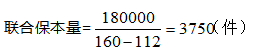A产品保本销售量=3750×3=11 250（件）

B产品保本销售量=3750×2=7500（件）

C产品保本销售量=3750×1=3750（件）

3.分算法。

假设固定成本按边际贡献的比重分配：A产品保本销售量=3750×3=11 250（件）

B产品保本销售量=3750×2=7500（件）

C产品保本销售量=3750×1=3750（件）

3.分算法。

假设固定成本按边际贡献的比重分配：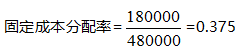分配给A产品的固定成本=240 000×0.375=90 000（元）

分配给B产品的固定成本=120 000×0.375=45 000（元）

分配给C产品的固定成本=120000×0.375=45 000（元）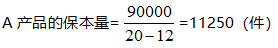A产品的保本额=11250×20=225000（元）

同理，B产品和C产品的保本量分别为7500件、3750件，它们的保本额分别为225 000元、150 000元。

233网校2019年中级会计模考大赛已开启，考前提分就现在>>加油包帮你再提20分！

2019年中级会计师辅导学习班，3科章节重难点、分值分布，以及复习策略全梳理，只需花费250+小时拿下机考核心考点，【点击听课>>

• 模拟试题38837试题

• 章节练习17369试题

• 离线做题不连网，流畅做题

省流量
• 历年真题2785试题

QQ群：110155092学霸集结，互动交流

相关推荐233网校微信公众号QQ群号：236715484